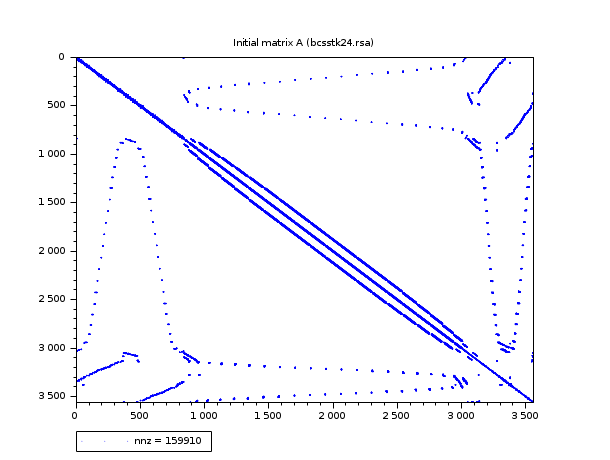Scilab Home page | Wiki | Bug tracker | Forge | Mailing list archives | ATOMS | File exchange
Please login or create an account
Change language to: English - Français - Português - Русский

Please note that the recommended version of Scilab is 6.0.2. This page might be outdated.
See the recommended documentation of this function

Scilab help >> UMFPACK Interface > taucs_chget

# taucs_chget

retrieve the Cholesky factorization at the scilab level

### Calling Sequence

`[Ct,p] = taucs_chget(C_ptr)`

### Arguments

C_ptr

a pointer to the Cholesky factorization (C,p : A(p,p)=CC')

Ct

a scilab sparse matrix (you get the upper triangle i.e. Ct is equal to C')

p

column vector storing the permutation

### Description

This function may be used if you want to plot the sparse pattern of the Cholesky factorization (or if you code something which use the factors). Traditionnaly, the factorization is written :

`P A P' = C C'`

with P' the permutation matrix associated to the permutation p. As we get the upper triangle Ct (= C'), in scilab syntax we can write :

`A(p,p) = Ct' * Ct`

### Examples

```// Example #1 : a small linear test system
A = sparse( [ 2 -1  0  0  0;
-1  2 -1  0  0;
0 -1  2 -1  0;
0  0 -1  2 -1;
0  0  0 -1  2] );
Cp = taucs_chfact(A);
[Ct, p] = taucs_chget(Cp);
full(A(p,p) - Ct'*Ct)  // this must be near the null matrix
taucs_chdel(Cp)```
```// Example #2 a real example
stacksize(3000000)  // the last PlotSparse need memory
// first load a sparse matrix
[A] = ReadHBSparse(SCI+"/modules/umfpack/examples/bcsstk24.rsa");
// compute the factorisation
Cptr = taucs_chfact(A);
// retrieve the factor at scilab level
[Ct, p] = taucs_chget(Cptr);
// plot the initial matrix
xset("window",0) ; clf()
PlotSparse(A) ; xtitle("Initial matrix A (bcsstk24.rsa)")
// plot the permuted matrix
B = A(p,p);
xset("window",1) ; clf()
PlotSparse(B) ; xtitle("Permuted matrix B = A(p,p)")
// plot the upper triangle Ct
xset("window",2) ; clf()
PlotSparse(Ct) ; xtitle("The pattern of Ct (A(p,p) = C*Ct)")
// retrieve cnz
[OK, n, cnz] = taucs_chinfo(Cptr)
// cnz is superior to the realnumber of non zeros elements of C :
cnz_exact = nnz(Ct)
// do not forget to clear memory
taucs_chdel(Cptr)```### See Also

• taucs_chfact — cholesky factorisation of a sparse s.p.d. matrix
• taucs_chsolve — solve a linear sparse (s.p.d.) system given the Cholesky factors
• taucs_chdel — utility function used with taucs_chfact
• taucs_chinfo — get information on Cholesky factors
• taucs_chget — retrieve the Cholesky factorization at the scilab level
• cond2sp — computes an approximation of the 2-norm condition number of a s.p.d. sparse matrix

### Comments

Add a comment:
Please login to comment this page.

 Report an issue << taucs_chfact UMFPACK Interface taucs_chinfo >>

 Scilab EnterprisesCopyright (c) 2011-2017 (Scilab Enterprises)Copyright (c) 1989-2012 (INRIA)Copyright (c) 1989-2007 (ENPC)with contributors Last updated:Tue Apr 02 17:37:33 CEST 2013